## Solar neutrinosdeutsch

In the centre of the Sun nuclear reactions are taking place, which are providing the energy the Sun has been emitting from its surface during the last 4.5 billion years. In these reactions electron neutrinos are created as well, which leave the Sun unimpeded and which are detected and counted in terrestrial experiments. All four detectors (presently being in operation receive only about 50% of the neutrino flux predicted by theoretical solar models. To explain this deficit one uses particle physics theories which predict the conversion of these electron neutrinos into the two other known "flavours" while they are on their way from the solar core into the detectors. These other flavours are neither produced in nuclear reactions nor can be detected by the present detectors; they are therefore lost and lead to the deficit. The conversion from flavour "1" into "2" (or "3") is determined by two quantities called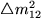and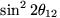(resp.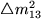and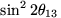), the values of which are as yet unknown. By computing a solar model, the number and energy spectrum of the electron neutrinos being emitted, the conversion into the two other neutrino flavours and finally by simulating the measurements of the incoming remaining electron neutrinos we are trying to determine the values for these four parameters, for which all three counting experiments can be explained simultaneously. In addition we try to reproduce the neutrino energy spectrum recently determined by Super-Kamiokande. Without this latter information the counting results can also be explained by a conversion into only one other flavour. From our results about the neutrino conversions elementary particle physicists gain additional insight into the structure of the smallest building blocks of matter.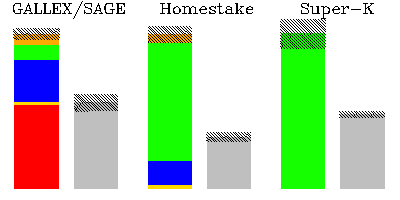Figure 1

Figure 1 shows predicted count rates for Solar neutrinos in the three experiments, GALLEX/SAGE, Homestake, Super-Kamiokande (coloured bars) in comparison with the actual number of counts detected (gray histograms). The hashed areas reflect the theoretical resp. experimental uncertainties in the rates. Since the neutrinos are created with different energies in the various nuclear reaction rates and the experiments have different energy sensitivities, the mixture of neutrinos from the various reaction sources (colour-coded in Fig.1) varies from experiment to experiment as seen in the theoretical prediction. For example, Super-Kamiokande can measure almost exclusively the most energetic neutrinos, while Gallium-experiments (GALLEX & SAGE) can see a mixture of all sources. The ratios of observed-to-predicted counts differ between the four experiments (although always being of order 50%); from this one can already deduce that the mechanism leading to the deficit is energy-dependent.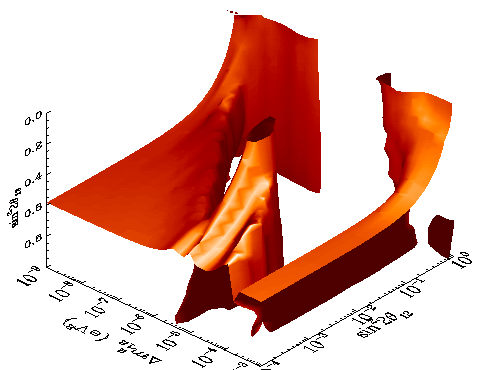Figure 2

The conversion of electron-neutrinos into the two other flavours 2 and 3 is determined by 4 quantities of unknown values. The above figure 2 shows those regions in the 3-dimensional subspace (the parameterhas been omitted to allow a graphic representation), for which our model reproduces approximately the number of measured neutrinos in all three types of experiments (Figure 1) by simulating the number of neutrinos created in the solar fusion reactions and the subsequent conversion into non-detectable flavours. Also reproduced is the neutrino energy spectrum (as measured by Super-Kamiokande). Regions of solutions are contained between the reddish surfaces. In case one of these regions runs parallel to an axis (e.g. the tube at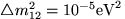and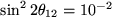) this means that the conversion properties into the third flavour are unimportant. However, such solutions are not very accurately reproducing all experimental results (see Figures 3 and 4).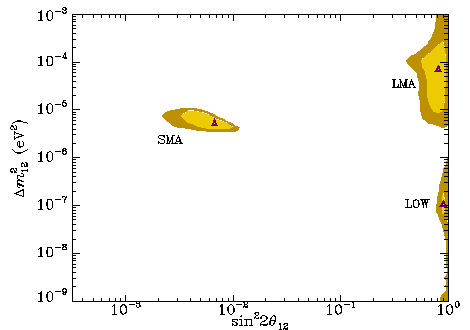Figure 3

To account for only the number of measured neutrinos (Figure 1) the conversion into only one other flavour is sufficient (see Figure 3). In this case, Figure 2 reduces to a plane in which the solutions are shown by coloured islands. The best solutions are marked by triangles.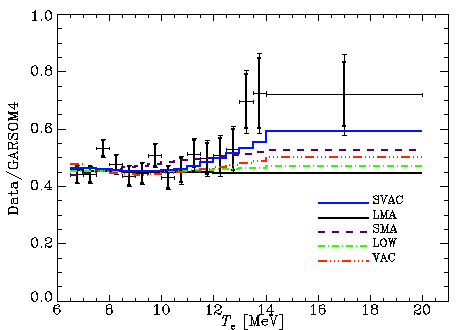Figure 4

This figure shows how different solutions of the neutrino counts (Figure 1) agree with the measured neutrino energy spectrum shown as symbols with error bars (actually, the energy spectrum of electrons is measured in Super-Kamiokande, which interact with the incoming neutrinos, on whose energies their own spectrum therefore depends). The solutions of Figure 3 cannot reproduce the increase at highest energies; for this, the additional conversion into the third flavour (see the SVAC solution) is necessary.

H. Schlattl, A. Weiss

• Where are the solar neutrinos?, H. Schlattl, Annual Report of the MPA, 1998, pp.7-9 (ps, pdf)

• Neutrino-Physik mit Hilfe der Sonne, A. Weiss, MPG-Jahrbuch 1999 (ps)

• A solar model with improved subatmospheric stratification, H. Schlattl, A. Weiss & H.G. Ludwig A&A 322 (1997), p.646

• Signatures of the efficiency of solar nuclear reactions in the neutrino experiments, H. Schlattl, A. Bonnano & L. Paterno, Phys.Rev.D, submitted (1999), Preprint astro-ph/9902354